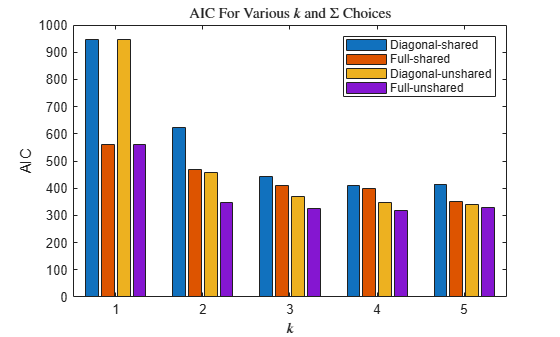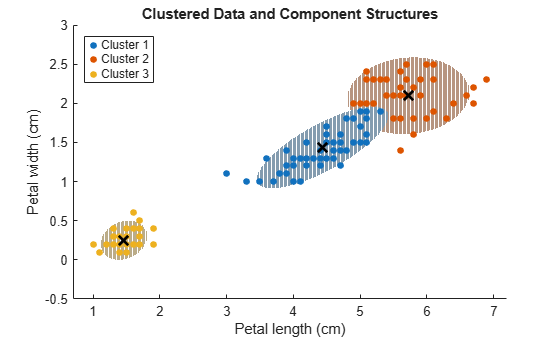# Tune Gaussian Mixture Models

This example shows how to determine the best Gaussian mixture model (GMM) fit by adjusting the number of components and the component covariance matrix structure.

Load Fisher's iris data set. Consider the petal measurements as predictors.

```load fisheriris; X = meas(:,3:4); [n,p] = size(X); rng(1); % For reproducibility figure; plot(X(:,1),X(:,2),'.','MarkerSize',15); title('Fisher''s Iris Data Set'); xlabel('Petal length (cm)'); ylabel('Petal width (cm)'); ```Suppose k is the number of desired components or clusters, andis the covariance structure for all components. Follow these steps to tune a GMM.

1. Choose a (k,) pair, and then fit a GMM using the chosen parameter specification and the entire data set.

2. Estimate the AIC and BIC.

3. Repeat steps 1 and 2 until you exhaust all (k,) pairs of interest.

4. Choose the fitted GMM that balances low AIC with simplicity.

For this example, choose a grid of values for k that include 2 and 3, and some surrounding numbers. Specify all available choices for covariance structure. If k is too high for the data set, then the estimated component covariances can be badly conditioned. Specify to use regularization to avoid badly conditioned covariance matrices. Increase the number of EM algorithm iterations to 10000.

```k = 1:5; nK = numel(k); Sigma = {'diagonal','full'}; nSigma = numel(Sigma); SharedCovariance = {true,false}; SCtext = {'true','false'}; nSC = numel(SharedCovariance); RegularizationValue = 0.01; options = statset('MaxIter',10000); ```

Fit the GMMs using all parameter combination. Compute the AIC and BIC for each fit. Track the terminal convergence status of each fit.

```% Preallocation gm = cell(nK,nSigma,nSC); aic = zeros(nK,nSigma,nSC); bic = zeros(nK,nSigma,nSC); converged = false(nK,nSigma,nSC); % Fit all models for m = 1:nSC; for j = 1:nSigma; for i = 1:nK; gm{i,j,m} = fitgmdist(X,k(i),... 'CovarianceType',Sigma{j},... 'SharedCovariance',SharedCovariance{m},... 'RegularizationValue',RegularizationValue,... 'Options',options); aic(i,j,m) = gm{i,j,m}.AIC; bic(i,j,m) = gm{i,j,m}.BIC; converged(i,j,m) = gm{i,j,m}.Converged; end end end allConverge = (sum(converged(:)) == nK*nSigma*nSC) ```
```allConverge = logical 1 ```

`gm` is a cell array containing all of the fitted `gmdistribution` model objects. All of the fitting instances converged.

Plot separate bar charts to compare the AIC and BIC among all fits. Group the bars by k.

```figure; bar(reshape(aic,nK,nSigma*nSC)); title('AIC For Various \$k\$ and \$\Sigma\$ Choices','Interpreter','latex'); xlabel('\$k\$','Interpreter','Latex'); ylabel('AIC'); legend({'Diagonal-shared','Full-shared','Diagonal-unshared',... 'Full-unshared'}); figure; bar(reshape(bic,nK,nSigma*nSC)); title('BIC For Various \$k\$ and \$\Sigma\$ Choices','Interpreter','latex'); xlabel('\$c\$','Interpreter','Latex'); ylabel('BIC'); legend({'Diagonal-shared','Full-shared','Diagonal-unshared',... 'Full-unshared'}); ```According to the AIC and BIC values, the best model has 3 components and a full, unshared covariance matrix structure.

Cluster the training data using the best fitting model. Plot the clustered data and the component ellipses.

```gmBest = gm{3,2,2}; clusterX = cluster(gmBest,X); kGMM = gmBest.NumComponents; d = 500; x1 = linspace(min(X(:,1)) - 2,max(X(:,1)) + 2,d); x2 = linspace(min(X(:,2)) - 2,max(X(:,2)) + 2,d); [x1grid,x2grid] = meshgrid(x1,x2); X0 = [x1grid(:) x2grid(:)]; mahalDist = mahal(gmBest,X0); threshold = sqrt(chi2inv(0.99,2)); figure; h1 = gscatter(X(:,1),X(:,2),clusterX); hold on; for j = 1:kGMM; idx = mahalDist(:,j)<=threshold; Color = h1(j).Color*0.75 + -0.5*(h1(j).Color - 1); h2 = plot(X0(idx,1),X0(idx,2),'.','Color',Color,'MarkerSize',1); uistack(h2,'bottom'); end h3 = plot(gmBest.mu(:,1),gmBest.mu(:,2),'kx','LineWidth',2,'MarkerSize',10); title('Clustered Data and Component Structures'); xlabel('Petal length (cm)'); ylabel('Petal width (cm)'); legend(h1,'Cluster 1','Cluster 2','Cluster 3','Location','NorthWest'); hold off ```This data set includes labels. Determine how well `gmBest` clusters the data by comparing each prediction to the true labels.

```species = categorical(species); Y = zeros(n,1); Y(species == 'versicolor') = 1; Y(species == 'virginica') = 2; Y(species == 'setosa') = 3; miscluster = Y ~= clusterX; clusterError = sum(miscluster)/n ```
```clusterError = 0.0800 ```

The best fitting GMM groups 8% of the observations into the wrong cluster.

`cluster` does not always preserve cluster order. That is, if you cluster several fitted `gmdistribution` models, `cluster` might assign different cluster labels for similar components.